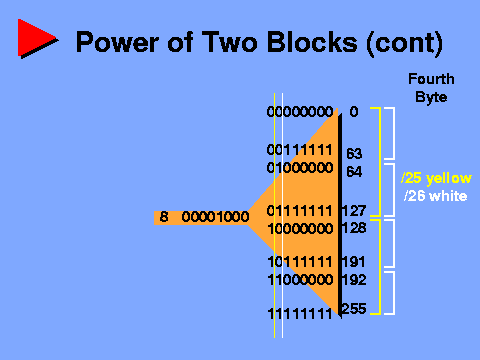Connected: An Internet Encyclopedia
Power of Two Blocks (cont)

Up: Connected: An Internet Encyclopedia
Up: Programmed Instruction Course
Up: Subnetting and CIDR
Prev: Power of Two Blocks
Next: Quiz 1Power of Two Blocks (cont) Increasing our prefix length beyond /24 moves us out of the third byte and into the upper bits of the fourth byte. /25, illustrated in yellow, causes each number in the third byte to be split into two ranges, one corresponding to 0-127 in the fourth byte, the other matching 128-255 there. So, 10.10.8.0/25 matches 10, 10 and 8 exactly in the first three bytes, and 0 through 127 in the fourth byte. 10.10.8.128/25 matches the same three numbers in the initial bytes, and 128 through 255 in the fourth byte. The next few address blocks at this prefix length would be 10.10.9.0/25, 10.10.9.128/25 and 10.10.10.0/25. Note that we are now working with blocks of 128, on boundaries of multiples of 128, now on the fourth byte.

Increasing the prefix length again we reach /26, shown in white. Now each of the numbers in the third byte breaks down into four address ranges, corresponding to 0-63, 64-127, 128-191 and 192-255 in the fourth byte. Now we have blocks of 64, on boundaries of multiples of 64.

To summarize: the IP address is a continuous string of 32 bits. Because it is written as four 8-bit decimal values, it can be confusing to visualize how various address prefixes fit together. Remember, byte boundary or not, every time you increase the prefix length by one and move the boundary one bit to the right, the number of matching blocks is doubled, but the size of each block is reduced by half. Conversely, decreasing the prefix length by one and moving the boundary one bit to the left groups pairs of blocks together, halves the total number of blocks, and doubles the size of each. Always start at the closest byte boundary and work the bits from there.

Exercise: I've illustrated the region around /24, the boundary between the third and fourth bytes, but it's important to remember that this diagram could be extended downward, to the left and to the right almost indefinitely. I suggest you try it for yourself - draw a diagram similar to this one, only illustrating the region around /16, the boundary between the second and third bytes. Mark the prefix blocks corresponding to /15, /14 and /13, then do /17 and /18. Pick some sample numbers, construct IP address prefixes, and determine which sets of addresses match.

Next: Quiz 1

Connected: An Internet Encyclopedia
Power of Two Blocks (cont)